[BACK]
 Intelligent Automation & Soft ComputingDOI:10.32604/iasc.2022.025395Article

PMSG Based Wind Energy Conversion System Using Intelligent MPPT with HGRSC Converter

University College of Engineering, Dindigul, Tamilnadu, India
Received: 22 November 2021; Accepted: 18 January 2022

Abstract: Wind power conversion systems play a significant position in grid-coupled renewable source networks. In this paper, a permanent magnet based synchronous alternator type wind energy scheme is considered for analysis. The enhanced performance of wind power conversion could be reached by improving maximum power point tracking (MPPT) and by modernising the control circuit of the power electronic circuit. The main task is to enrich its performance level by proposing fuzzy gain scheduling (FGS) based optimal torque management for maximum power point tracking. In addition to the improved MPPT, this article analyses different topologies of direct current–direct current (DC–DC) converters such as boost converter and high-gain resonant switched capacitor converter. Comparative simulation analysis of converters with conventional optimal torque control and proposed FGS-based optimal torque control using MATLAB/simulink are presented with various wind speeds.

Keywords: Wind power conversion scheme; permanent magnet type synchronous alternator; optimal torque; FGS based optimal torque control; high gain resonant switched capacitor converter

1  Introduction

Renewable type energy schemes have definitely expanded its commitment for creation of electrical source energy, that is harmless to the ecosystem, and limiting the reliance on petroleum product-based power generation technique . Among the sustainable energy creation frameworks, the wind energy conversion systems (WECS) are generally liked for their wide accessibility and improved innovation in the execution of large-sized wind turbines.

Extensively variable speed machine has prominent superiority beyond fixed type speed turbines namely enhanced energy capture, capable of operating in the maximum value power point, good efficiency and enriched power quality. In addition, synchronous generators with permanent magnets are generally utilised in WECS. To be sure, this procedure can supplant the field coil turn of a synchronous machine unit and contains more notable benefits of smaller size that include more power density, loss reduction, excellent reliability and robustness [2,3]. The applications of a permanent magnet synchronous generator (PMSG) are with a switch-mode rectifier that includes an uncontrolled alternating current (AC) to direct current (DC) converter (a full-bridge uncontrolled diode rectifier) and also a DC–DC type converter. The latter type is widely used because of its advantages. The benefits are generator and power converter simplicity, a minimum number of active switches, increased reliability and minimum cost of the entire system [4,5].

Extracting high power from a wind turbine machine is done by controlling the generator speed or torque, which is in turn done by controlling an interfaced DC to DC type converter. Many researchers analysed numerous MPPT techniques, for instance, tip speed ratio (TSR) , optimal torque control (OTC) , power signal feedback (PSF) control  and hill-climb searching (HCS) control . Among the various MPPT methods, optimal torque control is a simple, fast, and commonly used method . To enhance the efficiency of WECS, conventional OTC needs to be improved. Therefore, in this paper, improved OTC with an artificial intelligent fuzzy gain scheduling controller is incorporated.

DC/DC boost converter can accomplish boundless voltage change extents, yet in every practical sense the most limited increment is confined by circuit abandons for example the parasitic segments and switch replacement times . Also boost converter needs a high duty ratio to attain good voltage gain, which increases the size of passive components [13,14]. Parastar et al. 2015 analyzed , a high gain resonant switched-capacitor (RSC) converter device with noteworthy advantages, including a flexible assembly, reduced voltage stress and loss in the switches. In this article, the efficiency of a wide-speed wind energy conversion scheme is analysed with two converter topologies, namely the boost converter and the high gain resonant switched capacitor converter.

2  Proposed System

The planned wind energy system comprises of PMSG Wind speed Turbine with uncontrolled diode-rectifier, MPPT controlled DC to DC level converter, and also a grid side coupled inverter along with a control circuit is shown in Fig. 1.Figure 1: The schematic diagram of proposed scheme

2.1 Wind Turbine Machine

The mechanical energy is attained by using wind turbine, it converts the wind kinetic energy into mechanical energy. Horizontal axis wind turbine (HAWT) is considered in this section for its high effectiveness. Also, the machine tip-speed ratio (λ) is indicated by:

λ=ωmR/v  (1)

Here R indicates the blade length, ωm is rotor speed (in rad/s) and v indicates wind speed (in m/s). Mathematically the power produced by a wind-turbine is :

PTurbine=12ρπR2Cp(λ,β)v3 (2)

where, ρ points the density of air (kg/m3), R indicates radius of the blade (m), Cp shows the performance coefficient of the turbine and finally β indicates pitch angle of rotor blades (in degrees). Any variation in the wind speed or the generator speed initiates the adjustment in the machine tip speed ratio prompting power coefficient variety. Subsequently, extricated power is influenced.

2.2 PMSG

The PMSG is applied as a wind generator that changes over the mechanical energy into electrical energy. The electrical torque (Te) in terms of mechanical power (Pm) is presented in equation number (3) and the mechanical torque (Tm) is presented in the Eq. (4),

Te=Pm/ωr  (3)

Tm=0.5×Cρv3×60n2πng  (4)

where, ωr is rotor speed, n points the gearbox ratio and ng indicates the generator speed in revolution per minute (RPM) . The three-phase uncontrolled rectifier in sequence with the PMSG that accepts variable output voltage and converts into pure DC.

2.3 DC–DC Converter

Uncontrolled rectifier precedes DC–DC converter which converts AC output into DC. The DC–DC type converter increases the magnitude of output resultant voltage when compared to input. It is controlled using MPPT, that is used to track maximum value of power from a wind source generator. Meantime MPPT regulates DC coupled voltage. In this methodology, two types of DC–DC converters are analyzed.

2.4 MPPT

Optimal torque control MPPT is applied in this paper to extract maximum power from the generator. This method entails only a single active switching component, which is adapted to control and adjust the machine torque at its optimal values. This method is ease and economic solution to track maximum power.

2.5 Grid Side Coupled Converter (GSC) Device

The grid coupled converter scheme deals with regulated DC link voltage. The GSC device is a three-phase two-stage voltage type source inverter that converts DC link voltage into AC. Along with the DC–AC conversion GSC also controls reactive power. Switching devices in a GSC is controlled using GSC based controller.

Each segment of the intended structure is elaborated in the upcoming discussions.

3  Boost Converter

DC–DC boost converter is a voltage step-up converter, which boosts the magnitude of output voltage compare to input [18,19]. The key operating feature of a boost type converter device is the inductor present in input side of the circuit will oppose sudden changes of input current. Along these lines, this converter is appropriate to interface high load/battery voltages.

4  High Gain Two-Stage RSC Converter

Switched-capacitor (SC) DC to DC type converter has significant interest in high-power applications field because of its higher-power density, good efficiency and ease control. Parastar et al. (2015) analysed high gain RSC (HGRSC) for 10 MW WECS and validated its effectiveness in the aspect of efficiency. Certain windmills in the range of megawatts (MW) are expected to have interfere with high voltage (HV) type power schemes. To manage this circumstance a high gain proposed RSC designs can be acquainted to achieve a very good high voltage gain and maximum evaluated power . Two modular cells are main parts of RSC converter that chooses a novel array of diodes, solid-state device switches, inductors and resonant capacitors. The configuration for High gain two-stage RSC converter is shown in Fig. 2.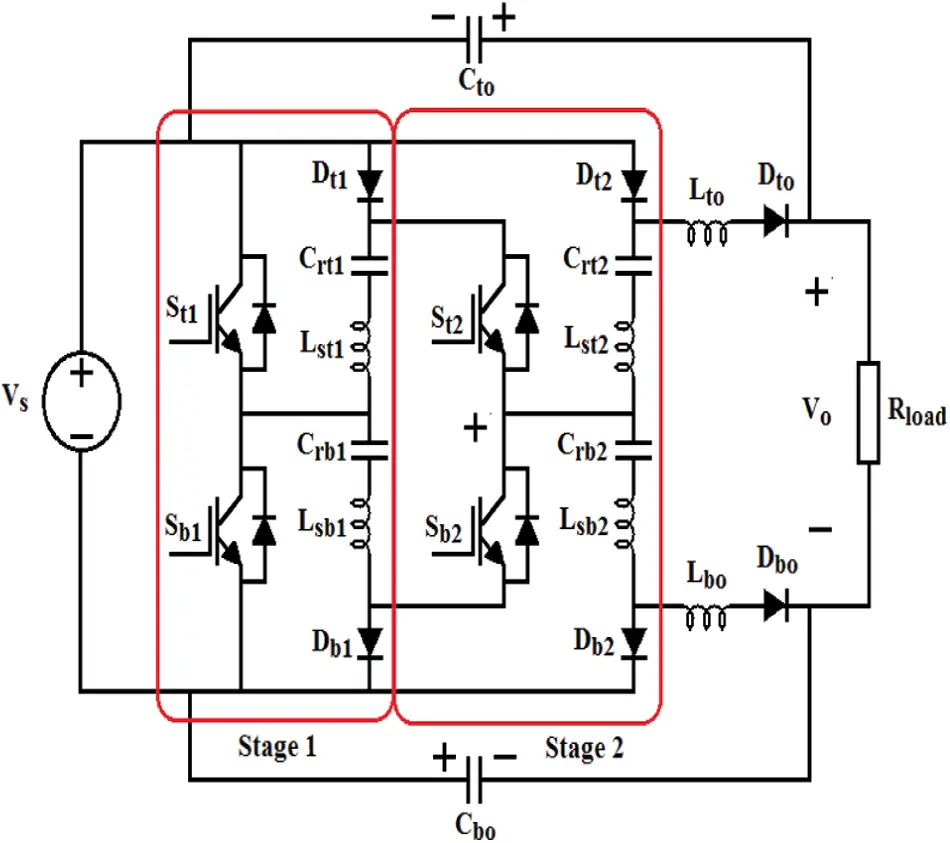Figure 2: RSC converter with 7 levels

Also Fig. 2 displays a 7-level RSC device. The RSC system comprises of four resonant capacitors and inductors and a couple of output filter capacitors and resonant inductors with source voltage Vs and output voltage Vo. Also, it consists of active devices of six diodes, and four switches (St1, St2, Sb1 and Sb2). The switching devices (St1, St2) and (Sb1, Sb2) are activated complementarily to mitigate the conduction losses in active and passive devices.

The converter is operated under four sequences such as

•   Bottom switching devices are made ON, while top switching devices are held OFF

•   The whole active devices are turned OFF during this time

•   The top-level switches in this arrangement are made ON, while lower or bottom switching devices are switched OFF

•   Entire active devices are turned OFF in this time period

When anyone of segment devices is turned ON then voltage level of the output capacitors were charged upto thrice of the input voltage. This stored voltage is discharged to load when all the devises are turned OFF.

VO=(2k+11)VS (5)

It is observed from Eq. (5) that the examined k-stage resonant switched capacitor converter naturally supports tremendous gains by the most un-number of gadgets due to its exponential effect. In this article k is 2, to get maximum voltage gain of 7 as per Eq. (5). It is observed from the discussion, turn OFF time (duty ratio) decides the voltage discharge. The proposed configuration maximum efficiency is limited with the duty ratio of 0.5 . Simulink model of proposed converter is shown in Fig. 3.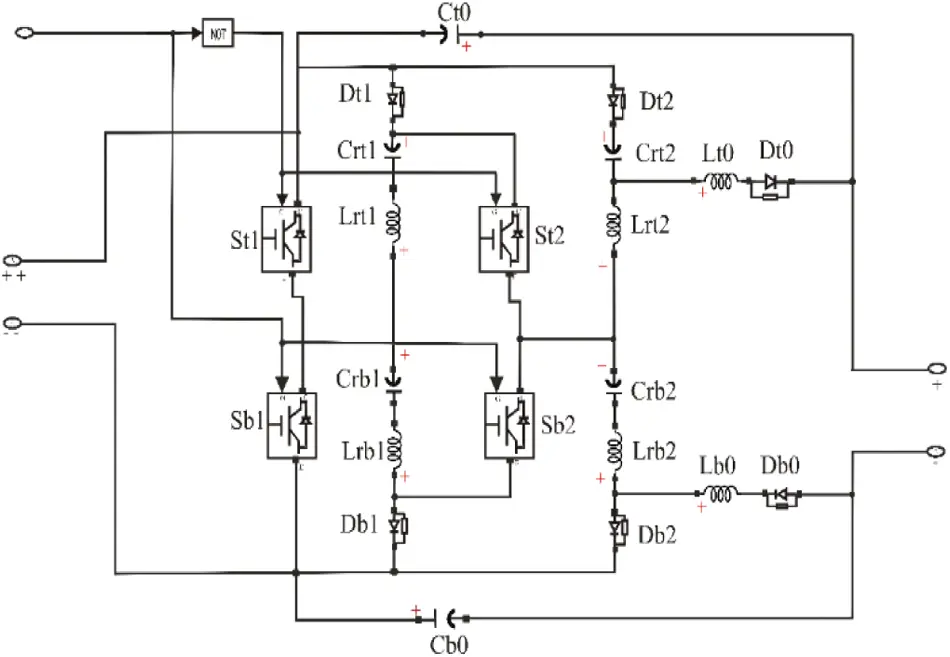Figure 3: Simulink model of proposed seven levels RSC

5  Optimum Torque Control

In view of the fluctuating behavior between the wind and wind turbine power curve, then to track extreme power the generator torque or speed must be balanced in response to the speed of wind. The OTC technique controls the machine torque and tunes it for an optimum value at any wind speed conditions. The mechanical power Pm is:

Pm=0.5ρACρvω3=0.5ρACρ(ωmRλ)3  (6)

In an Eq. (6) A is the circular area covered by blade. For an assumed wind turbine characteristic, the optimal tip speed ratio is specified. Consequently, the optimal mechanical angular velocity of a rotor is presented as (7):

ωm_opt=λoptRvω=Kωvω  (7)

The optimum mechanical power Pm_opt is found by Eq. (8):

Pm_opt=0.5ρACρ_opt(Rωm_optλopt)3=Kopt(ωm_opt)3  (8)

Additionally, optimum mechanical torque is determined as below:

Tmopt=Kopt(ωm_opt)2  (9)

Therefore, steps for OTC procedure are recognized as follows:

1)   Initially it senses generator speed ωg.

2)   Then estimate the reference torque Tg by using generator efficiency ηg in (10):

Tg=ηgKopt(ωg)2 (10)

3)   After that the reference torque from step 2 is utilized to estimate the dc reference current along with measurement of the rectifier output voltage Vdc1 by using given Eq. (11):

Idc1=(Tg.ωg)Vdc1  (11)

4)   The error value from the reference dc current and measured dc current is applied together for varying the duty cycle of the switching device to get a regulated output from the DC–DC converter and also the generator torque regulated by means of a proportional integral (PI) controller.

5.1 PI Controller in OTC

The PI controller has the property of a forward regulator that is hugely incorporated in different utility of the power network . Also, the steady state error is hold down efficiently by utilizing the PI controller. The OTC type PI controller deals with the DC current error (ec) to produce duty cycle (d). The expression for PI controller in OTC is

d= Kpec+Kicedt (12)

Both gains of controller (Kp & Ki) are tuned by Ziegler-Nichols’ method. Since Ziegler-Nichols’ method gains of controller are not an optimal under variable wind speed, in this analysis soft computing tuning of PI controller is discussed in following sections.

5.2 Fuzzy Gain Scheduling Controller in OTC

The unchanging gains of the PI controller for change in an input error produce an abrupt change in duty cycle. The soft computing tuning of Kp and Ki in a PI controller can defeat this issue. Since Kp and Ki are the parameters which decides the PI controller output (duty ratio), fine tuning is necessary in OTC. In this evaluation the fuzzy gain scheduling controller is proposed for the auto tuning of gains . Fuzzy logic controllers (FLCs) are suitable for nonlinear system and system with vague data . Their advantages are non-requirement of a mathematical model, robustness, and acceptance of nonlinearity [23,24]. In optimum torque control case, it gets input as DC current error in order to produce the values of Kp and Ki as output. The principle of designing fuzzy rules is that the output of the controller can make the system output response dynamic and static performances optimal. In Mamdani class of fuzzy the min-max scheme of fuzzification is employed. The Centroid technique of defuzzification is practiced for its accuracy, to achieve the output .

Tabs. 1 and 2 displays the laws for both KP and Ki.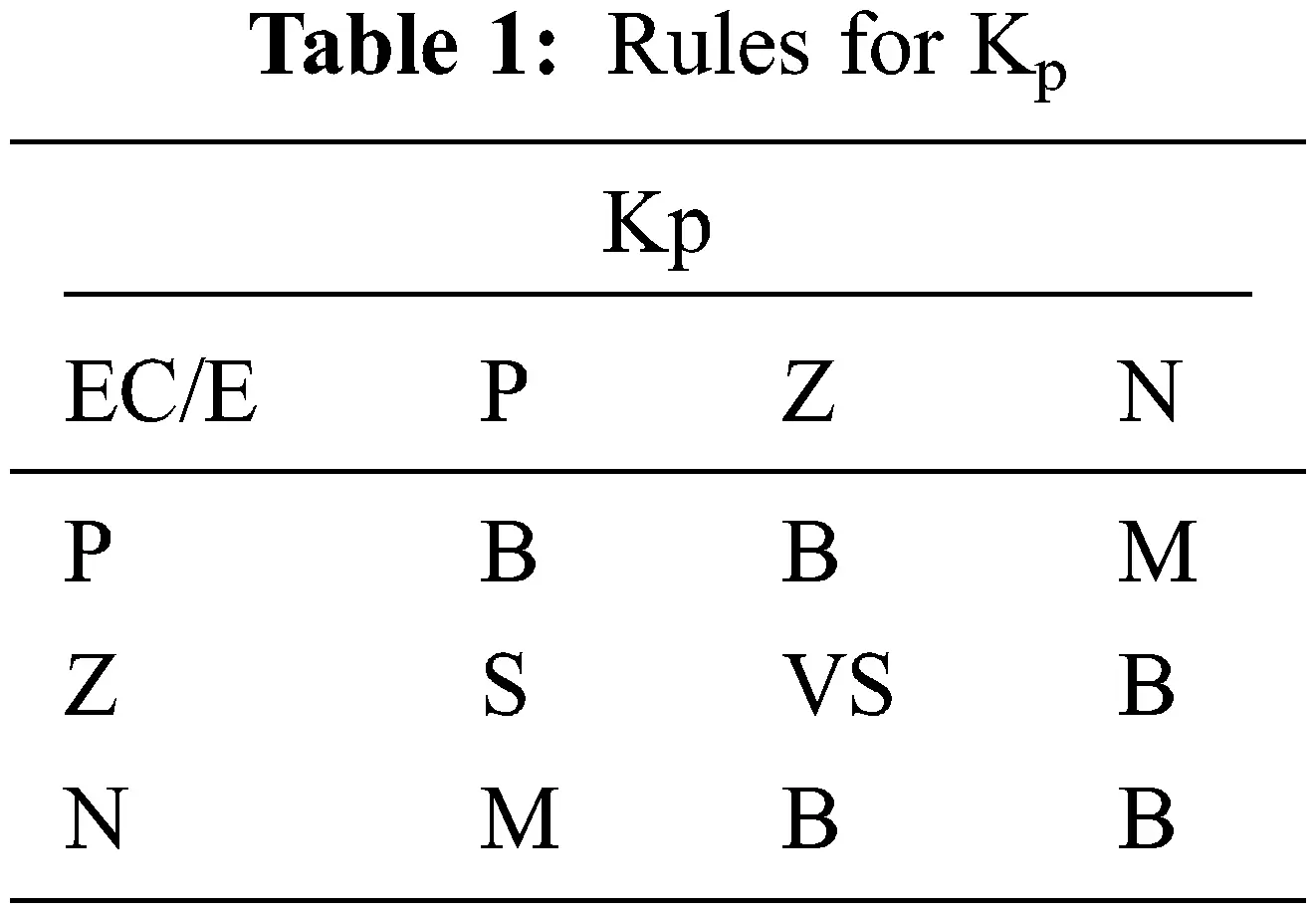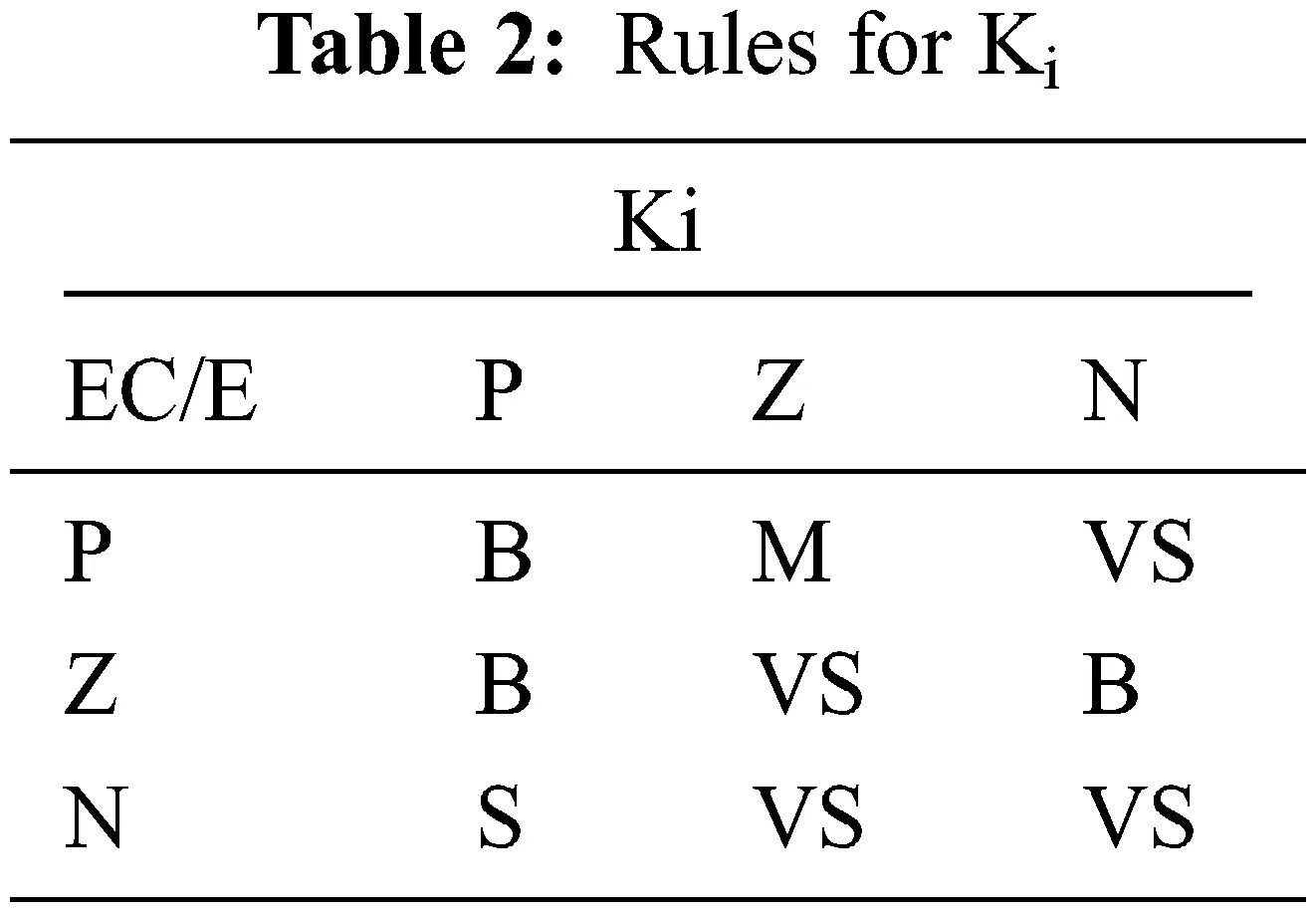The laws in the FGS PI controller gives results for the output dependency on input, but the various rules of a controller finalize the execution period in real-time application. Ananth Moorthy et al. (2013) analysed PMSM control using FGS with various number of rules like 25, 16 and 9, which validates the efficacy of 9 rules . Here, 9 least number of rules referred is applied. In the proposed article the current error (E) and change in error (EC) are used as inputs for controller with membership functions Positive, zero, Negative {P, Z, N}. Also, Kp and Ki are the outputs obtained with the membership functions very small, small, medium, big {VS, S, M, B}. The Fig. 4 displays the membership function of E and EC. Fuzzy gain scheduling controller reduces the error in DC link voltage.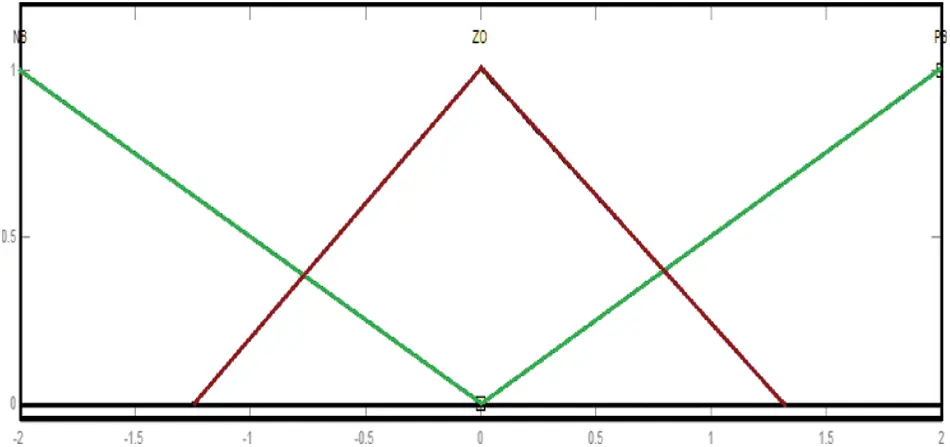Figure 4: Membership functions of two inputs E and EC

6  Grid Coupled Converter Supervisory Act

The grid coupled converter supervises the reactive power and the DC link potential vdc. Phase locked loop tracks grid system voltage vector and generates angle Θg . The grid coupled converter control arrangement is shown in Fig. 5. Here d-axis from synchronous edge is associated with the grid with voltage vector to attain a voltage-oriented control (VOC) scheme, hence (vdg = vg) and q-axis voltage vg becomes zero, from where the reactive and active power values obtained using :

Pg=32vdgidg  (13)

Qg =32vdgiqg  (14)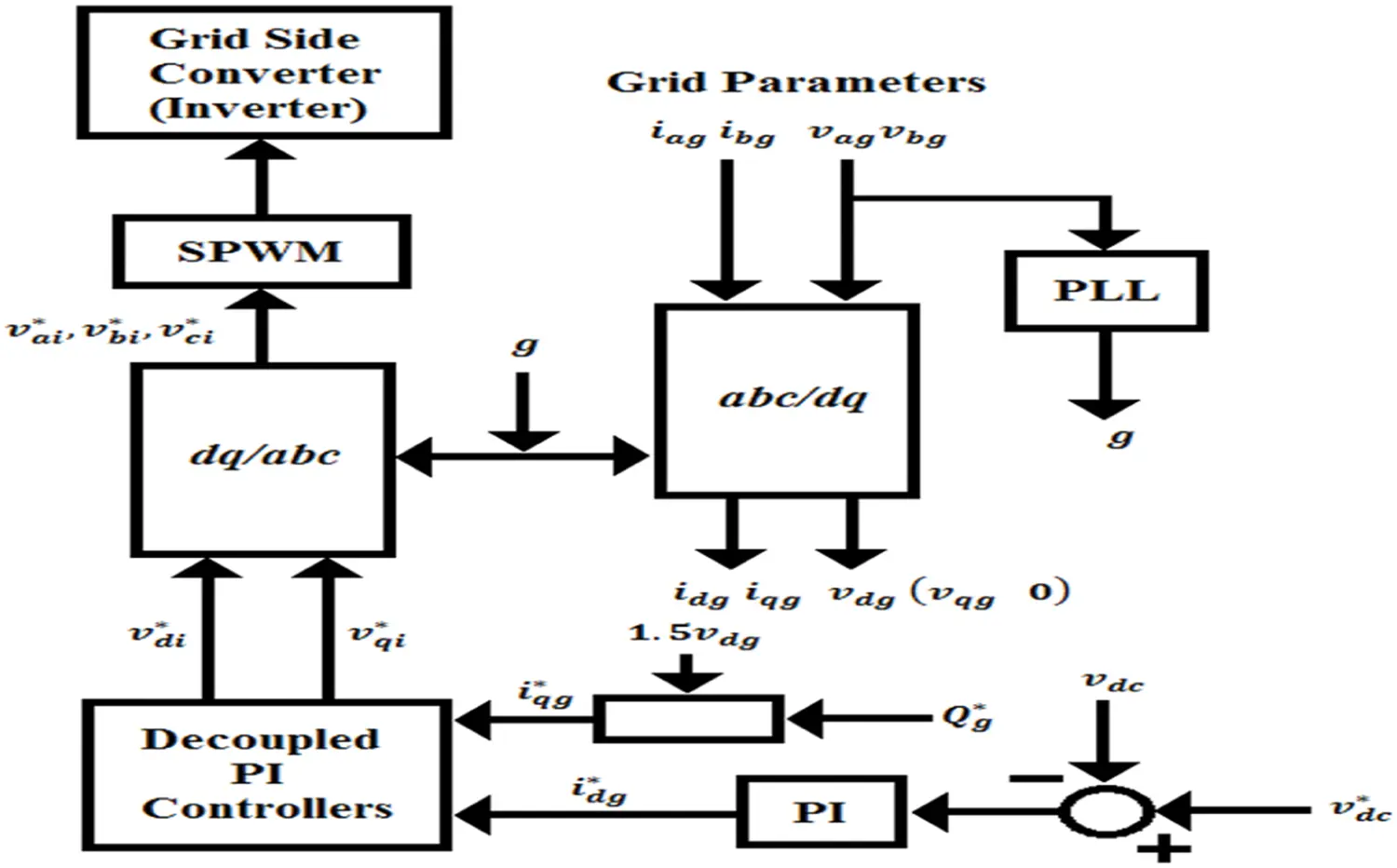Figure 5: Grid side converter control scheme

The reference current value for q-axis could be achieved through

iqg=23Qgvdg  (15)

Considering inverter losses are negligible, then real power of AC inverter is

Pg=32vdgidg=vdcidc  (16)

The DC reference voltage can be obtained from

vdc=6Vailma  (17)

where Vail is inverter line voltage and ma is the modulation index to control sinusoidal pulse width modulation (SPWM) to trigger the inverter.

7  Simulation Results and Analysis

In this analysis, two cases of wind speeds are considered to analyze the performance of WECS using PI based OTC with boost converter, PI and FGSPI based OTC with HGRSC converter.

Case 1: In this case, a fixed wind speed value is fixed as 12 m/s to study the performance of a projected system. Performance analysis of PI controlled HGRSC converter based WECS under case 1 is shown in Fig. 6.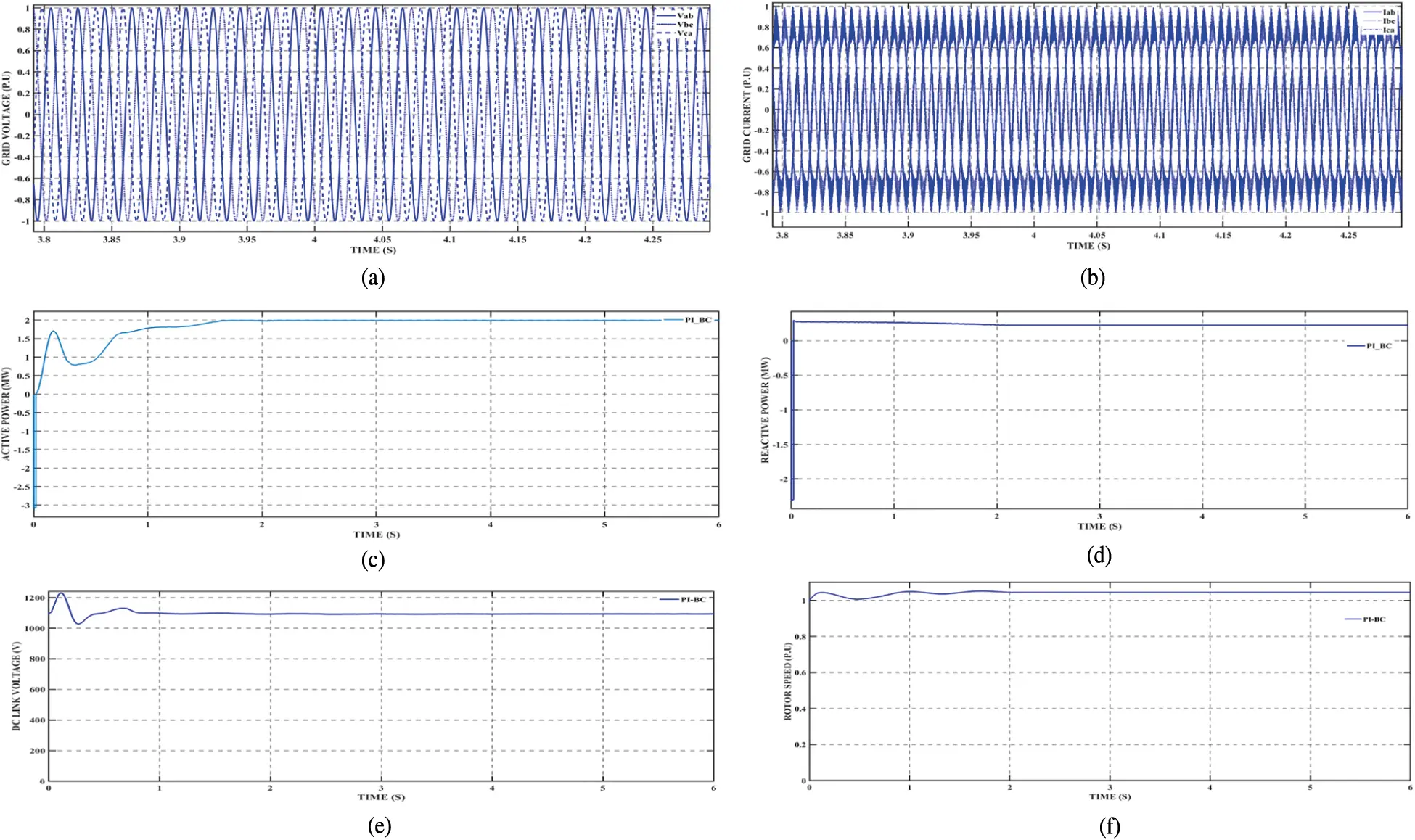Figure 6: Performance of PI controlled boost converter based WECS at constant wind speed (a) Grid voltage (b) Grid current (c) Power response (Active) (d) System's power (reactive) (e) DC linked potential (f) Rotor speed

In Fig. 6, it is observed that the system is simulated at a speed (wind) of 12 m/s. It shows that the rotor speed is slightly varied till 2 s after that rotor speed of the system is a constant which is 1.0454 P.U since the wind movement speed is maintained at constant value. With this rotor speed, the grid voltage and current of is system produced as 0.9987 and 0.9986 P.U. When rotor speed becomes 1.0454 P.U, the DC link voltage 1095 V is produced by the boost converter with an active power of 1.995 MW and maintained constantly. It is also noted that the reactive power is 0.27 MW at the starting, after 2 s the power is reduced to 0.2235 MW.

Performance analysis of PI controlled HGRSC converter based WECS under case 1 is displayed in Fig. 7.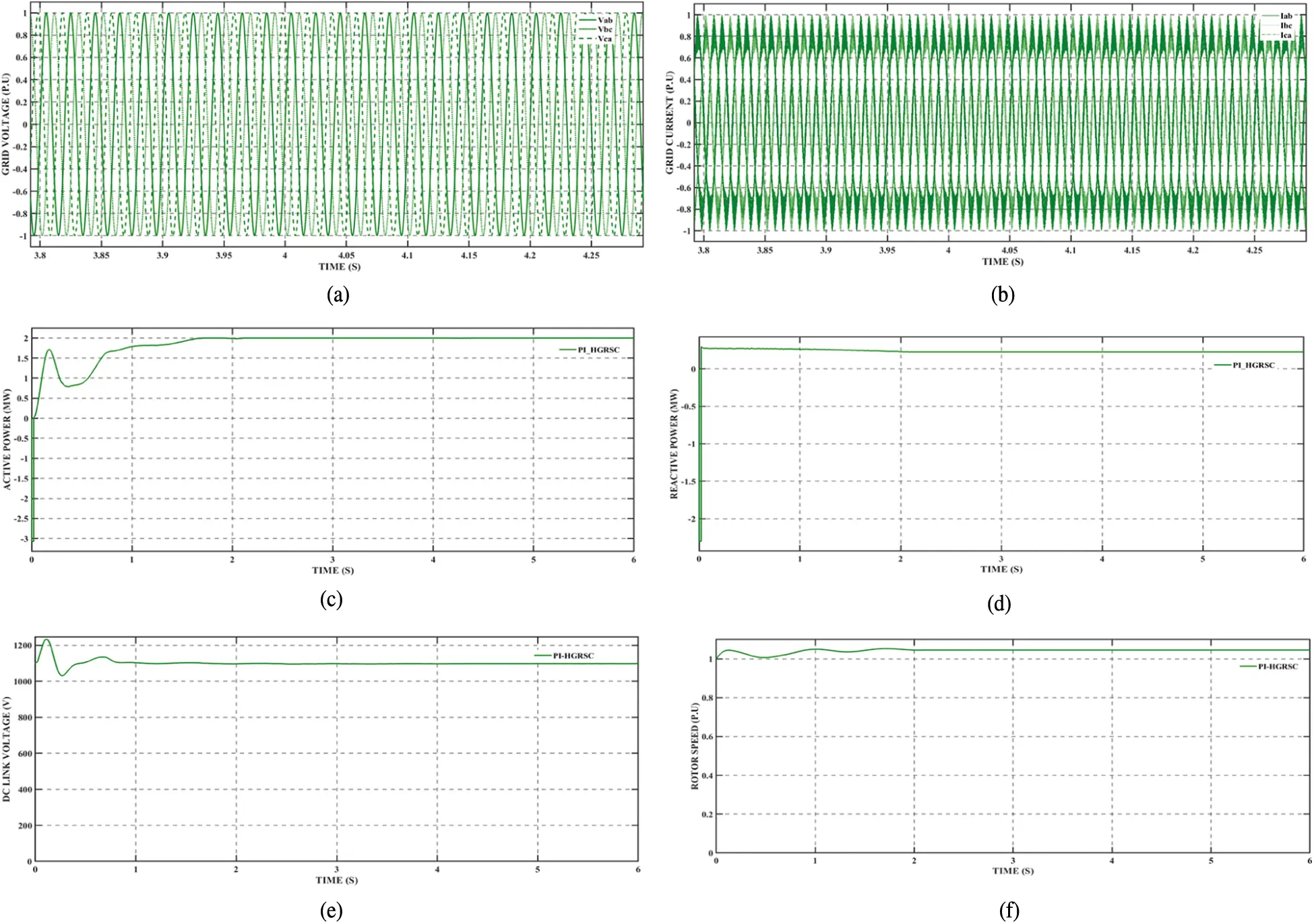Figure 7: Performance of PI controlled HGRSC converter based WECS with constant wind speed (a) Grid coupled voltage (b) Grid current (c) The power response (Active) (d) The reactive type power (e) DC linked voltage (f) Rotor speed

From Fig. 7, it noted that the slight variations in the rotor speed till 2 s after that rotor speed is constantly 1.0454 P.U.

In this particular rotor speed, the DC linked voltage 1097 V is generated by HGRSC which found to be slightly higher than the voltage achieved in the boost converter. The grid connected voltage and also current of the system are found to be 0.9992 and 0.9991 P.U. Furthermore, the reactive power is 0.27 MW initially, after that the power is sustained to 0.2236 MW. Similarly, there is an insignificant variation of active power at the beginning, but after 2 s it is found to be 1.997 MW and constantly maintained.

Performance analysis of FGS-PI controlled HGRSC converter based WECS under case 1 is shown in Fig. 8.Figure 8: Performance of FGS-PI controlled HGRSC converter based WECS with constant wind speed (a) Grid coupled potential (b) Current at grid (c) The power response (Active) (d) The reactive power response (e) DC coupled voltage (f) Speed of machine (rotor)

Fig. 8 shows that all considered parameters values are improved well by using FGS. The DC link voltage of 1100 V generated by the FGS-PI controlled HGRSC, which is greater than the voltage produced by PI-boost converter and PI-HGRSC. The grid voltage and current are 1 and 1 P.U. Initially, the reactive power is noted as 0.27 MW which is same as the above two cases, after 2 s the power is 0.224 MW which is lesser than all other cases. The active power is also greater than the above cases.

Case 2: In this case, variable wind speed is considered for analysis. From 0 to 4 s wind speed is 12 m/s, then it is changed to 10 m/s.

Performance analysis of PI type-controlled boost converter based WECS under case 2 is shown in Fig. 9.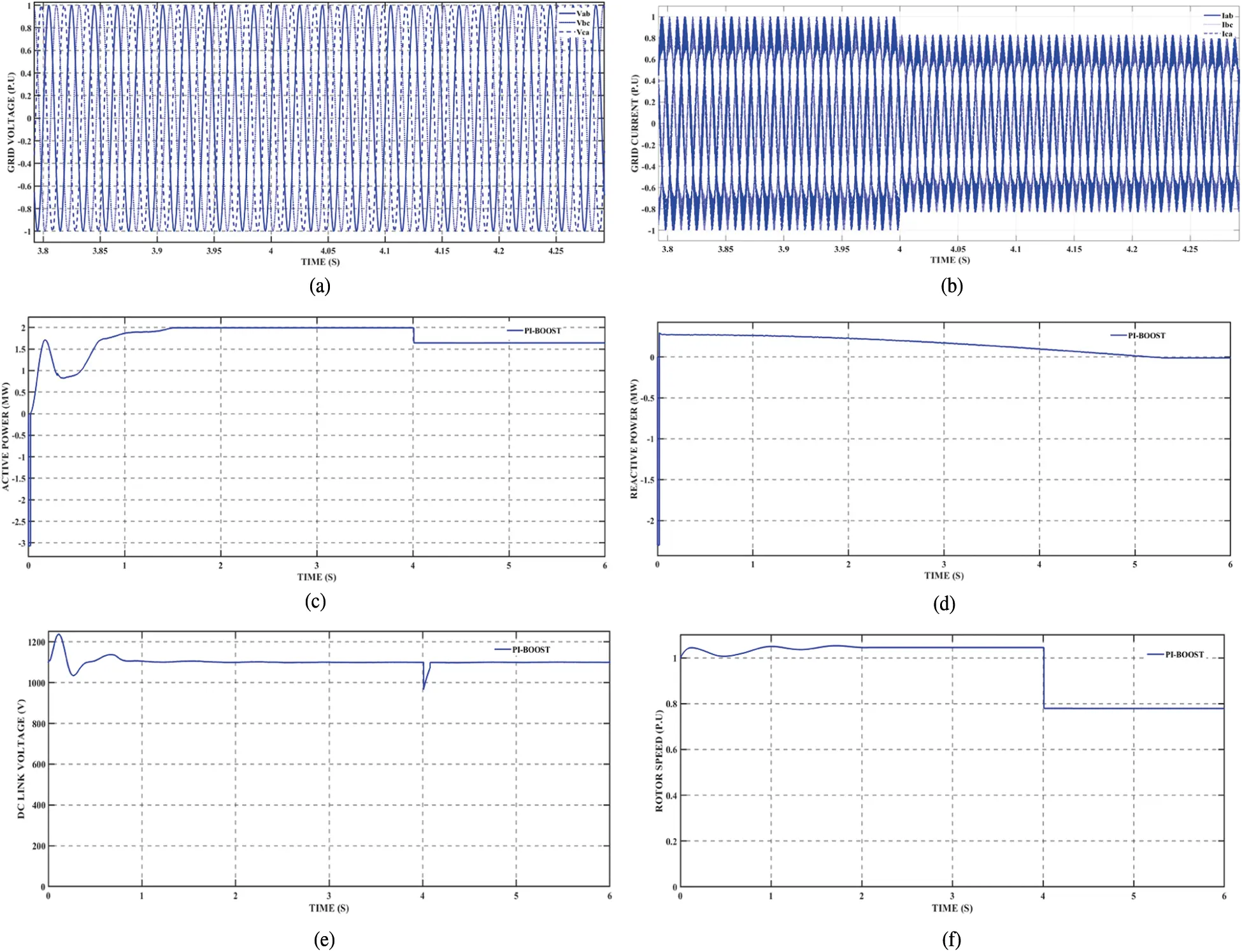Figure 9: Performance level of PI controlled boost converter based WECS at variable wind speed (a) Grid connected voltage (b) Current in grid (c) Power response (Active) (d) Reactive power response (e) DC linked voltage (f) Speed of machine (Rotor)

In this case, speed of wind is maintained at 12 m/s till 4 s after that it is dropped to 10 m/s. This sudden drop affects the rotor speed causing it to reduce the speed from 1.0454 to 0.77935, as displayed in Fig. 9.

The DC link supply 1100 V is maintained up to 4 s at which the speed of wind drops to 10 m/s, and also the potential drops to 965 V and finally the converter level up the voltage and settles the voltage to 1096 V at 4.1 s which is few volts lesser than voltage that occurred before reduction of wind speed.

At 12 m/s grid current is 1 P.U, after the drop in windy speed the grid current is reduced to 0.8312 P.U but the grid voltage is maintained constant even after windy speed drops i.e., 0.9987 V. Furthermore, the reactive power is 0.27 MW initially, after the drop in windy speed the power is sustained to 0.12 MW. Active power level is 1.995 at 12 m/s but after 4 s power is reduced and oscillates between 1.642 and 1.643 MW.

Performance analysis of PI controlled HGRSC converter based WECS under case 2 is shown in Fig. 10.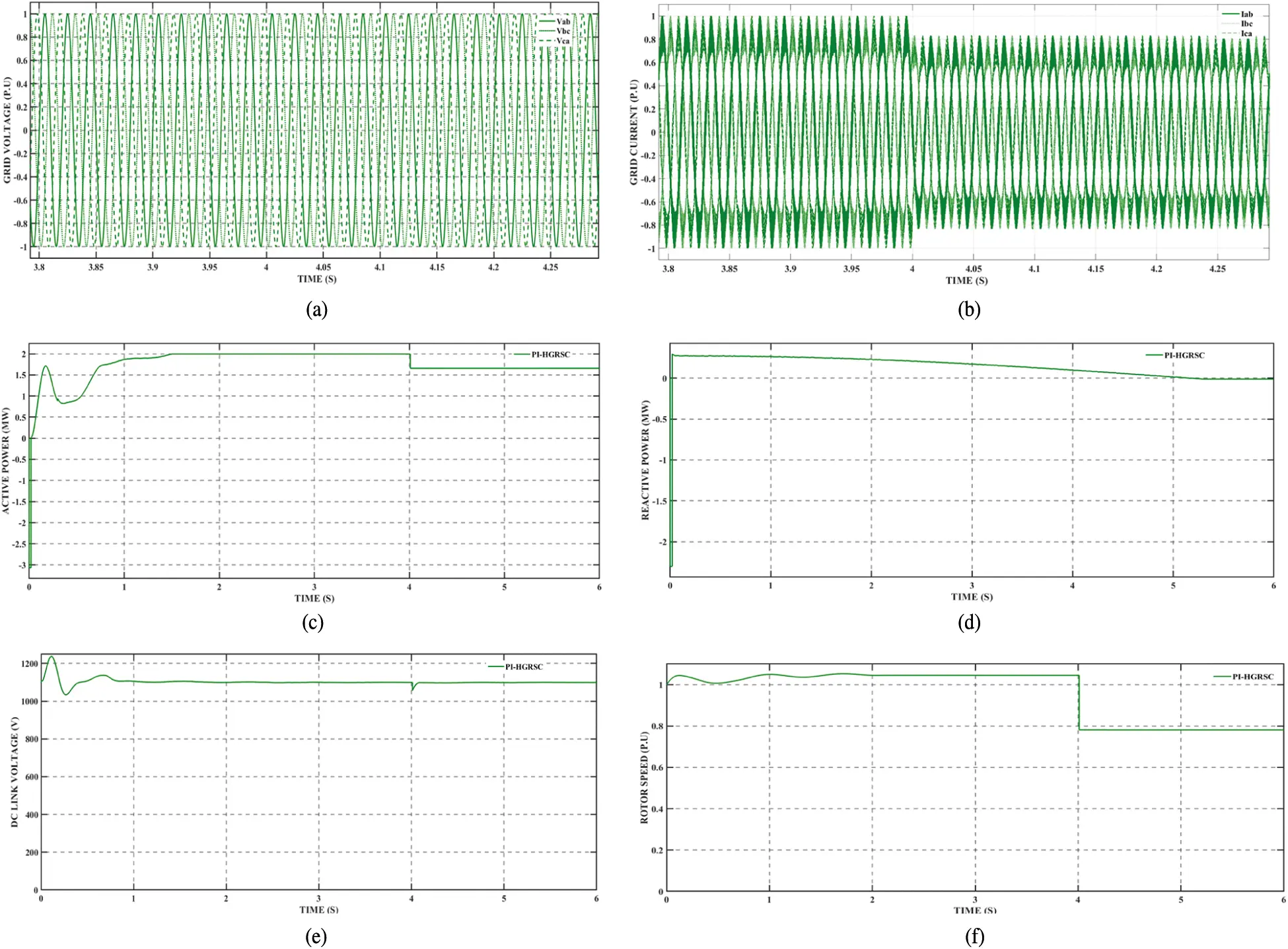Figure 10: Performance level of PI controlled HGRSC converter based WECS at variable wind speed (a) Grid connected voltage (b) Grid current (c) Active power response (d) Reactive power response (e) DC linked voltage (f) Rotor speed of machine

Fig. 10 shows that the DC link voltage is 1100 V at 12 m/s and as soon as wind speed drops to 10 m/s the voltage also drops to 1054 V. After that voltage gets gradually increased to 1098 V and settles at time 4.1 s which is comparatively lesser than voltage before the drop in wind speed but it has improved performance than the boost converter in the above case.

Similarly, the grid current at 12 m/s 1 P.U, after the drop in speed of wind causes the grid current is reduced to 0.832 P. U but the grid voltage is followed at a constant value i.e., 0.9992 P. U which is found to be greater than the above case. As same as the above case, reactive power is 0.27 MW initially, after the drop in windy speed the power is 0.12 MW. Also, Active power is 1.997 at 12 m/s but after 4 s the power is reduced and oscillates between 1.658 and 1.657 MW which is also greater than the above case.

Performance analysis of FGS-PI controlled HGRSC converter based WECS under case 2 is displayed in Fig. 11.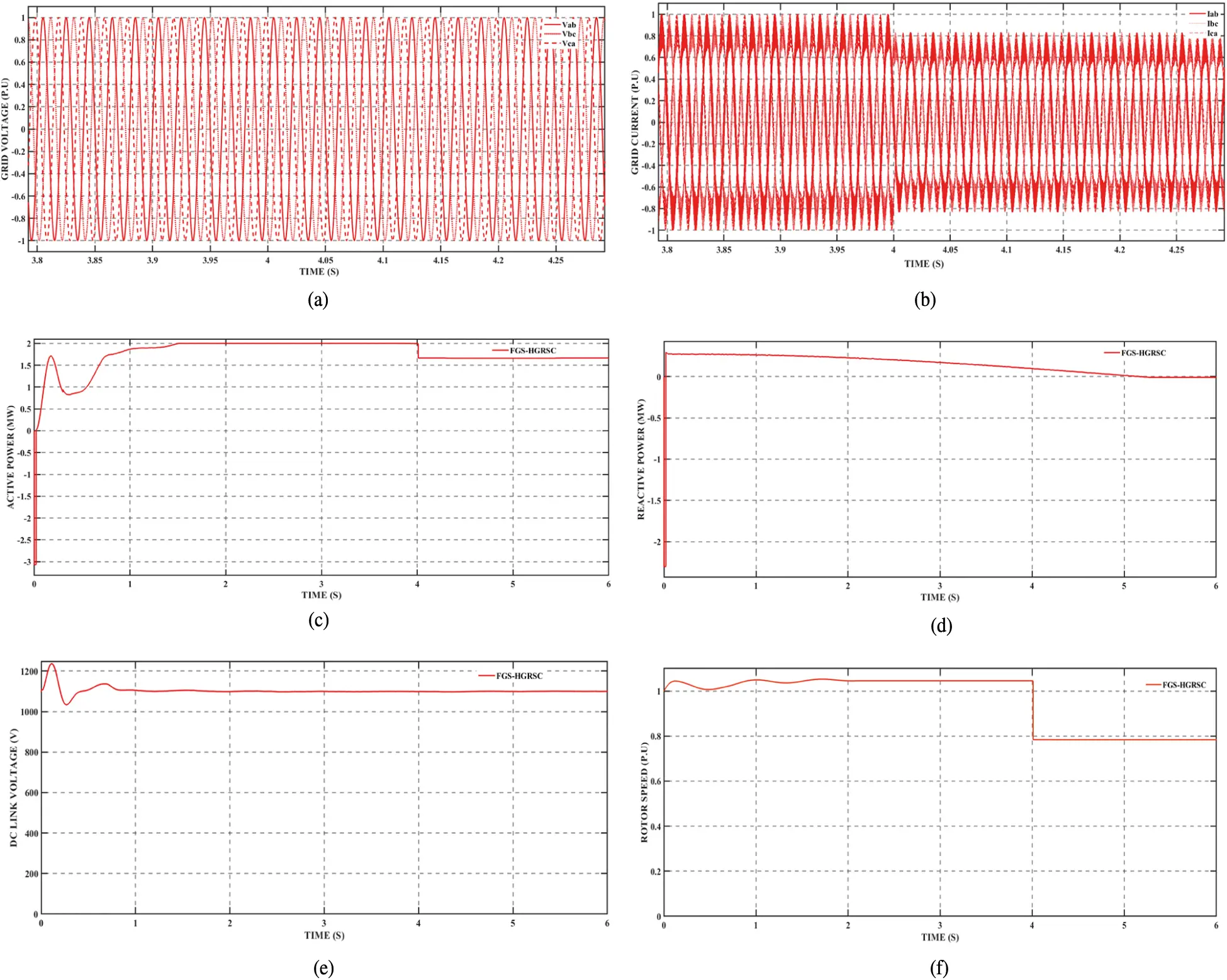Figure 11: Performance of FGS-PI controlled HGRSC converter based WECS at variable wind speed (a) Grid connected supply voltage (b) Current in grid (c) Power response (Active) (d) Reactive power response (e) DC linked voltage (f) Speed of machine (Rotor side)

From Fig. 11, it is detected that the dc linked voltage is 1100 V at 12 m/s and further as speed of wind drops to 10 m/s then the voltage also drops to 1097 V. From that FGS-HGRSC gradual increase of voltage to 1099 V is achieved and finally settles the voltage at time 4.07 s. This shows that the FGS-HGRSC has improved performance and settles the voltage rapidly than the above discussed case.

The grid current at 12 m/s 1 P.U, after the drop in wind speed the grid current is reduced to 0.8333 P. U, but the grid connected voltage is maintained constant, i.e., 1 P.U which is comparatively better than the above cases. Furthermore, the reactive power is 0.275 MW at 12 m/s, the power is reduced to 0.1 MW as the wind speed drops.

Also, Active power is 2 P.U at 12 m/s, but after the drop in wind speed power is reduced and gradually increased, which settled to 1.66 MW at 4.32 s but in other two cases the reactive power is oscillating and it does not settle down.

Performance analysis of WECS under case 2 is shown in Tab. 3.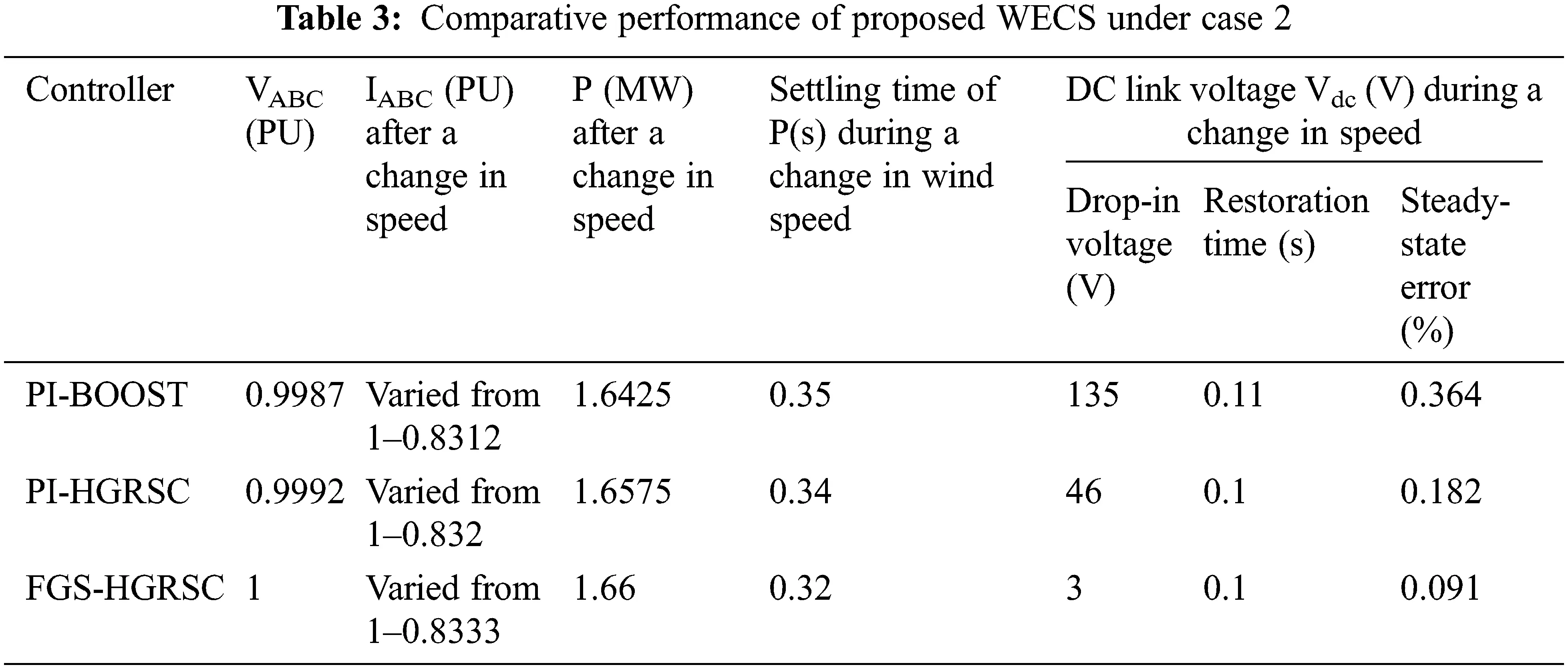From the Tab. 3, it is observed that grid voltage is maintained constant in all topologies even under change in wind speed. PI-boost and PI-HGRSC produces grid voltage of 0.9987 and 0.9992 P. U. Whereas FGS-HGRSC produces voltage of 1 P.U. In all configurations current produced is 1 P.U under wind speed of 12 m/s, when wind speed is reduced to 10 m/s current is also reduced to 0.8312, 0.832 and 0.8333 P. U by PI-boost, PI-HGRSC and FGS-HGRSC respectively. Drop-in DC link voltage Vdc (V) during a change in speed by PI-boost and PI-HGRSC are 135 and 46 V while FGS-HGRSC produces 3 V.

PI-boost and PI-HGRSC result Steady-state error in DC link voltage are 0.364% and 0.182%. FGS produces steady-state error of 0.091% which shows that soft computing method results less than 0.1% of steady state error by its effective control. After 4 s, active power produced by PI-boost, PI-HGRSC and FGS-HGRSC are 1.6425, 1.6575 and 1.66 P. U respectively. Tab. 3 shows that FGS-HGRSC has improved performance than the other two configurations.

8  Conclusion

This article discusses performance enhancement of the wind energy conversion system through improving MPPT and DC–DC converters on the generator side. In this paper, fuzzy gain scheduling controller-based optimal torque control MPPT is propounded. Enhanced working performance of the proposed method (MPPT) is presented with the comparative analysis of the convention OTC MPPT. Furthermore, the analysis is extended with improved converter methodology, namely a resonant switched capacitor converter with high gain. The wind energy conversion system using a boost converter and the HGRSC are analysed under constant and variable wind speeds. The resultant output of the proposed scheme is analysed in terms of DC-linked voltage, real power, reactive power and grid connected voltage. Under constant wind speed, this scheme delivers 5 kW greater than PI-based OTC with boost enhancer, but it is 3 kW higher than PI-based OTC with HGRSC converter. Under variable wind speeds, when wind speed decreases, PI-based OTC with each converter produces oscillating power. Furthermore, the proposed system offers steady power of 22 kW, which is higher than conventional systems. In the case of variation in wind speed, conventional OTC results in oscillation of DC linked voltage. DC linked voltage oscillation in the HGRSC converter is less than the boost converter based WECS, while the proposed MPPT with the HGRSC converter results in negligible fluctuation in voltage. From the analysis of both wind speeds, the results are noted that this FGS based OTC with HGRSC converter-based system offer improved performance than all other systems.

Funding Statement: The authors received no specific funding for this study.

Conflicts of Interest: The authors declare that they have no conflicts of interest to report regarding the present study.

## References

1. P. Veeramanikandan and S. Selvaperumal, “A fuzzy-elephant herding optimization technique for maximum power point tracking in the hybrid wind-solar system,” International Transactions on Electrical Energy Systems, vol. 30, no. 3, pp. e12214, 2020.
2. L. Barote, C. Marinescu and M. N. Cirstea, “Control structure for single-phase stand-alone wind-based energy sources,” IEEE Transactions on Industrial Electronics, vol. 60, no. 2, pp. 764–772, 201
3. M. H. Jafari Nadoushan and M. Akhbari, “Optimal torque control of PMSG-based stand-alone wind turbine with energy storage system,” Journal of Electric Power & Energy Conversion Systems, vol. 1, no. 2, pp. 52–59, 2016.
4. H. Polinder, J. A. Ferreira, B. B. Jensen, A. B. Abrahamsen, K. Atallah et al., “Trends in wind turbine generator systems,” IEEE Journal of Emerging and Selected Topics in Power Electronics, vol. 1, no. 3, pp. 174–185, 2013.
5. R. S. Semken, M. Polikarpova, P. Röyttä, J. Alexandrova, J. Pyrhönen et al., “Direct-drive permanent magnet generators for high-power wind turbines: Benefits and limiting factors,” IET Renewable Power Generation, vol. 6, no. 1, pp. 1–8, 2012.
6. J. Adhikari, I. V. Prasanna, G. Ponraj and S. K. Panda, “Modeling, design, and implementation of a power conversion system for small-scale high-altitude wind power generating system,” IEEE Transactions on Industry Applications, vol. 53, no. 1, pp. 283–295, 201
7. M. Yin, W. Li, C. Y. Chung, L. Zhou, Z. Chen et al., “Optimal torque control based on effective tracking range for maximum power point tracking of wind turbines under varying wind conditions,” IET Renewable Power Generation, vol. 11, no. 4, pp. 501–510, 2016.
8. M. A. Abdullah, A. H. M. Yatim, C. W. Tan and R. Saidur, “A review of maximum power point tracking algorithms for wind energy systems,” Renewable and Sustainable Energy Reviews, vol. 16, no. 5, pp. 3220–3227, 2012.
9. S. M. R. Kazmi, H. Goto, H. J. Guo and O. Ichinokura, “A novel algorithm for fast and efficient speed-sensorless maximum power point tracking in wind energy conversion systems,” IEEE Transactions on Industrial Electronics, vol. 58, no. 1, pp. 29–36, 2010.
10. N. Mohan., T. M. Undeland and W. P. Robbins, “Practical converter design considerations,” in The Power Electronics: Converters, Applications, and Design, 3rd edition, John Wiley & sons, USA, 2003.
11. I. Laird and D. D. C. Lu, “High step-up DC/DC topology and MPPT algorithm for use with a thermoelectric generator,” IEEE Transactions on Power Electronics, vol. 28, no. 7, pp. 3147–3157, 2012.
12. S. Selvaperumal, C. C. A. Rajan and S. Muralidharan, “Stability and performance investigation of a fuzzy-controlled LCL resonant converter in an RTOS environment,” IEEE Transactions on Power Electronics, vol. 28, no. 4, pp. 1817–1832, 20
13. S. Muralidharan, P. Nedumal Pugazhenthi, G. Prabahkar, J. Bastin Solai Nazren et al., “Performance investigation of SHE PWM implementation of GA based LCL resonant inverter in marine applications,” Indian Journal of Geo Marine Sciences, vol. 46, no. 9, pp. 1889–1898, 2017.
14. S. Selvaperumal and C. C. A. Rajan, “Micro-controller based LCC resonant converter analysis, design and simulation results,” International Journal of Computer and Electrical Engineering, vol. 1, no. 3, pp. 1793–8163, 2009.
15. A. Parastar and J. K. Seok, “High-gain resonant switched-capacitor cell-based DC/DC converter for offshore wind energy systems,” IEEE Transactions on Power Electronics, vol. 30, no. 2, pp. 644–656, 20
16. T. Pidiitia and G. T. R. Dasb, “Power maximization and control of PMSG wind energy system without wind speed sensors,” International Journal of Control Theory and Applications, vol. 10, no. 25, pp. 253–260, 2017.
17. C. Wei, Z. Zhang, W. Qiao and L. Qu, “An adaptive network-based reinforcement learning method for MPPT control of PMSG wind energy conversion systems,” IEEE Transactions on Power Electronics, vol. 31, no. 11, pp. 7837–7848, 2016.
18. M. Ellappan and K. Anbukumar, “Comparative analysis of ACM and GPWM controllers in continuous input and output power boost PFC converter,” Journal of Control Engineering and Applied Informatics, vol. 22, no. 4, pp. 61–70, 2020.
19. A. Marikkannan, B. V. Manikandan and M. Kumar, “ZVS asymmetrical PWM full-bridge high voltage gain DC–DC converter controlled by ANFIS for energy harvesting applications,” Journal of Control Engineering and Applied Informatics, vol. 21, no. 4, pp. 51–58, 20
20. I. Atawi and S. Zaid, “Model predictive control of H7 transformerless inverter powered by PV,” Intelligent Automation and Soft Computing, vol. 31, no. 1, pp. 449–46, 2022.
21. S. Ahmad, S. Ali and R. Tabasha, “The design and implementation of a fuzzy gain-scheduled PID controller for the festo MPS PA compact workstation liquid level control,” Engineering Science and Technology, an International Journal, vol. 23, no. 2, pp. 307–315, 2020.
22. T. Chaiyatham and I. Ngamroo, “Optimal fuzzy gain scheduling of PID controller of superconducting magnetic energy storage for power system stabilization,” International Journal of Innovative Computing, Information and Control, vol. 9, no. 2, pp. 651–666, 2013.
23. S. Selvaperumal and C. Rajan, “Investigation of fuzzy control based LCL resonant converter in RTOS environment,” Journal of Intelligent & Fuzzy Systems, vol. 26, no. 2, pp. 913–924, 2014.
24. P. Veeramanikandan and S. Selvaperumal, “Investigation of different MPPT techniques based on fuzzy logic controller for multilevel DC link inverter to solve the partial shading,” Soft Computing, vol. 25, no. 4, pp. 3143–3154, 2021.
25. J. J. Saade and H. B. Diab, “Defuzzification methods and new techniques for fuzzy controllers,” Iranian Journal of Electrical and Computer Engineering, vol. 3, pp. 161–174, 2004.
26. N. P. Ananth Moorthy and K. Baskaran, “Speed and torque control of permanent magnet synchronous motor using hybrid fuzzy proportional plus integral controller,” Journal of Vibration and Control, vol. 21, no. 3, pp. 563–579, 2013.This work is licensed under a Creative Commons Attribution 4.0 International License, which permits unrestricted use, distribution, and reproduction in any medium, provided the original work is properly cited.